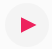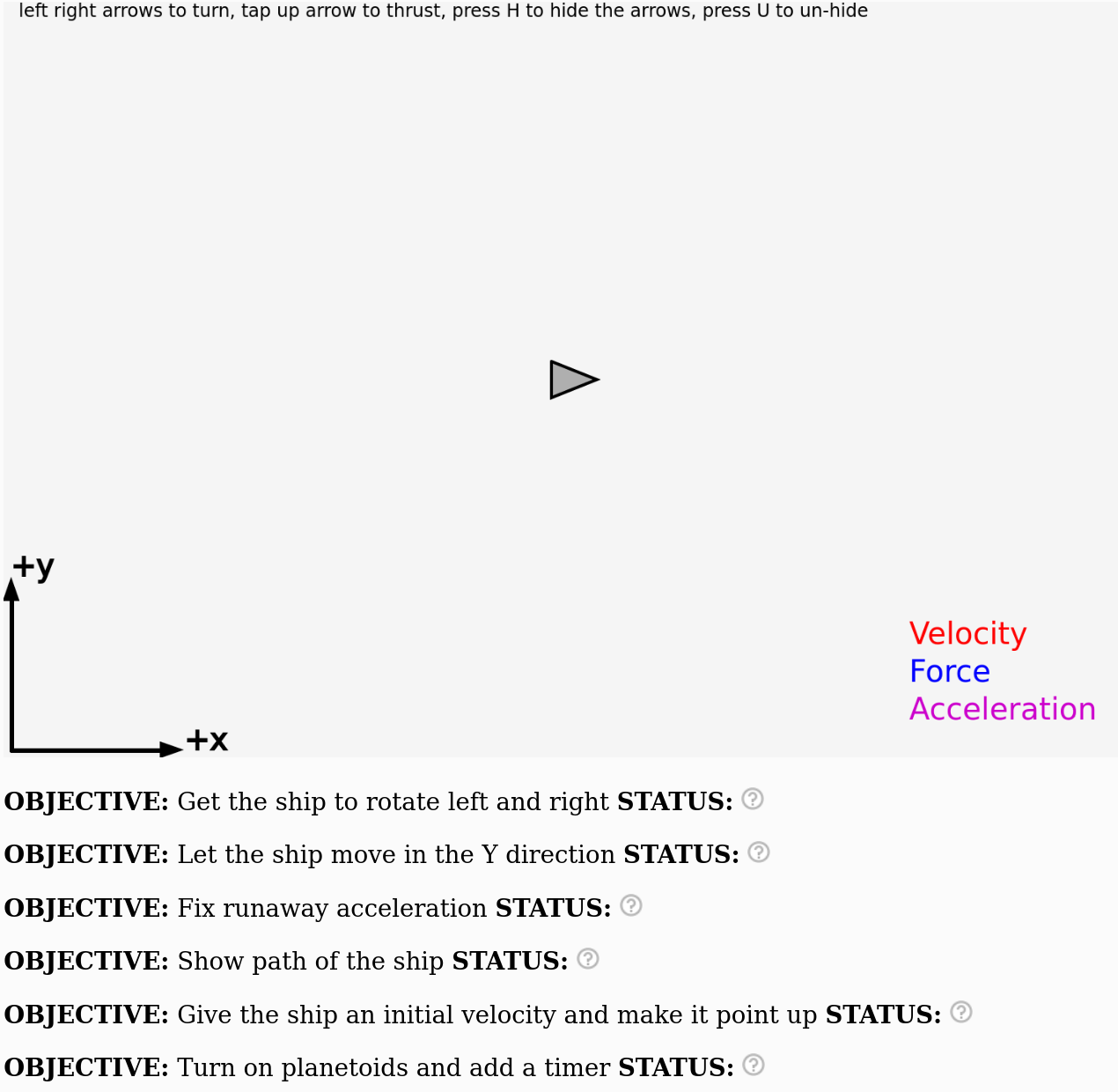# The Planetoids Game

This lab will guide you through coding up a web-based implementation of the classic video game asteroids. You will need to bring your laptop to the lab or use the available computers. Unfortunately, tablets will probably not work very well unless you have an external keyboard.

This example will use a programming language that is very similar to C and C++ programming. (Note: If you are familiar with C or C++ the main difference you will see is that there is no main() function and instead the draw() function serves this role.)

In the classic game of Asteroids, there is a little ship that flies around in space, very far away from stars and planets so that the gravitational acceleration is zero.

### Step 1. Play around with a 1-dimensional version of Asteroids

#### Very Important: Sign up for an account! Then click "Duplicate" so you can have your own version of the code!!!

In the top left corner, pressand you'll see something like this:Push up arrow to see the rocket accelerate. Unfortunately right now the ship can only accelerate in one direction. We will change this step-by-step to make the behavior more similar to the classic asteroids game. If the example doesn't work tell the instructor.

After you press all four arrow keys you will notice there are a couple of objectives that have not yet been completed:If the program can detect that something is wrong with your code it will show a rednext to that objective.

If the program can detect that you have completed the objective it will show a green check marknext to that objective.

But the program is not very smart and if it can't tell whether you have completed the objective it will indicate a question mark like thisnext to that objective.

By the end of this activity your goal is to have green check marks next to all the objectives like this:### Step 2. Look at the code

This is the code used to move the ship around in 1D version. Take a close look at the code and try to get an idea of what each part does:

function draw() {

// Update velocities
vx += deltaVx;

// Update location
x += vx*dt;

// velocity is unchanged if there are no forces
deltaVx = 0;

if (keyIsDown(LEFT_ARROW)) {
theta += 0.0;
}
if (keyIsDown(RIGHT_ARROW)) {
theta += 0.0;
}
if (keyIsDown(UP_ARROW)) {
// Rockets on!
accelx = Fthrust*cos(theta)/mass;
deltaVx = accelx*dt;
}
if (keyIsDown(DOWN_ARROW)) {
// Do nothing
}
if (keyIsDown(32)){ //spacebar
// Do nothing!
}

// Draw ship and other stuff
display();

} // end draw()   DO NOT ADD ANY CODE AFTER THIS LINE!!!


Any thoughts on what this means and what each part is doing?

### Step 3. Edit the source code to let the ship rotate!

After you click duplicate! to make sure that you're working on your own version of the code, change these lines of code:

    if (keyIsDown(LEFT_ARROW)) {
theta += 0.0;
} else if (keyIsDown(RIGHT_ARROW)) {
theta += -0.0;

to look like this:
    if (keyIsDown(LEFT_ARROW)) {
theta += 0.1;
} else if (keyIsDown(RIGHT_ARROW)) {
theta += -0.1;


The asteroids game is now set up so so that only the x-component of the thrust is what accelerates the ship. Notice that allowing the ship to rotate means that we can speed up and slow down the ship because we can rotate the rocket and apply a force that is opposite to the velocity vector. Our asteroids game is already a lot more fun! For reference, the game should now behave like this.

### Step 4. Enable multi-dimensional space travel (i.e. let the ship move in the y direction too)

Now take the code you've written and modify it so that the ship can move in the y direction. It will take a few different changes to get it to work.

#### Step 4a. Change the "Update location" section

Look at the "Update location" section. Right now it looks like this:

// Update location
x += vx*dt;


As you can see, only the x position is updated. The y position is not updated. Change the "Update location" section to this:

// Update location
x += vx*dt;
y += vy*dt;


#### Step 4.b. Change the "Update velocities" section

If you did step 4a correctly, we can now update both the x position and the y position, but only if $v_x$ and $v_y$ are non-zero! This will only happen if we change the "Update velocities" section so that both vx and vy are updated.

Change this:

\\ Update velocities
vx += deltaVx;


to this:

\\ Update velocities
vx += deltaVx;
vy += deltaVy;


#### Step 4.c. Objects in motion move in a straight line unless acted on by a force

Newton realized that objects move in a straight line with constant velocity if there are no forces acting on the object. For our computer program, we need to make sure that the velocity only changes when we push buttons on the keyboard. If we don't push any buttons then the change in velocity ($\Delta v_x$ and $\Delta v_y$) had better be zero.

This is the logic behind this part of the code:

    // velocity is unchanged if there are no forces
deltaVx = 0;


Change this to make sure deltaVy is set to zero too:

    // velocity is unchanged if there are no forces
deltaVx = 0;
deltaVy = 0;


This is an important step because if you do the next step correctly, but you forget to do this the ship will accelerate uncontrollably.

#### Step 4.d. Make sure that acceleration works in the y-direction

If you've done all the steps up to this point correctly, the ship still won't move in the y direction because we haven't told the ship how to accelerate in the y direction when the thrusters are turned on. Nowhere in the program do we set deltaVy equal to anything except zero!

Look closely at this part of the code:

    if (keyIsDown(UP_ARROW)) {
// Rockets on!
accelx = Fthrust*cos(theta)/mass;
deltaVx = accelx*dt;
}


You'll need to change this to something like this:

    if (keyIsDown(UP_ARROW)) {
// Rockets on!
accelx = Fthrust*cos(theta)/mass;
deltaVx = accelx*dt;
accely = ????
deltaVy = accely*dt;
}


It's your job to replace the ???? with something that actually gives the correct acceleration in the y direction. (Hint: it is very similar to the code for accelx but the trig function might be different! Should you use cosine, sine or tangent for the trig function? If you're not sure just try one and see what happens!)

### Step 5. Check that everything works as expected!

If you make all these changes your code should behave like this and your ship should be able to move in 2 dimensions!

Potential pitfall: Which trig function did you use to determine the y-component acceleration?

### Step 6. Add code to show the path of the ship

After display(); you can add this code and it will show the path of the ship:

    for( i = 0; i < xhistory.length ; i+= 1) {
drawPoint(xhistory[i],yhistory[i]);
}


### Step 7. Give the ship an initial velocity

Change the ship so that it comes in from the left with an initial velocity. Modify the beginning of the code to this:

x = 0;
y = 250;

vx = 25;
vy = 0;


and just for fun change the angle of the ship so that it points straight up:

theta = 3.141/2;


As you can see, the angle is being changed from 0 to $\pi/2$ radians. If converted to degrees this would be 90 degrees. This should make the ship point straight up (click here if curious about why).

### Step 8. Fire the thrusters and look closely at the trajectory of the ship

Let the ship drift a little bit and then fire the thrusters. How does the trajectory (the curve) look?

Play around with the ship for 2-3 minutes! Change the initial velocity, position and angle of the ship. See what happens!

Near the beginning of the code add this line:

draw_planetoids = true;


If you add this to your code the program should behave like this

Note: later you can turn the planetoids off with this code:

draw_planetoids = false;


Also go ahead and set the initial velocity back to zero vx = 0; vy = 0; and initial position back to  x = 375; y = 250;

### Step 10. Find the best value of the mass of the ship to survive!

Change the value for the mass until you find the best choice to survie the longest in the asteroid field. Which value for mass do you think is the best?

Optional: If you want to keep track of how much time has elapsed before you die you can add this code to the beginning:

    t = 0;


And then put this code after the display(); function but not before the end of the draw function:

     t += dt;
drawText('time = ',0.75*width,0.75*height);
drawText(t,0.85*width,0.75*height);


## Challenges: Choose one!

This is one of the easier challenges. Notice that your code has a section for when you press the down arrow:

    if (keyIsDown(DOWN_ARROW)) {
// Do nothing
}


Where it says "Do nothing" how would you add code to fire the thrusters in reverse?

Hint: You want to accelerate in the opposite direction of the acceleration you get when the thrusters are turned on.

### Option: Add a projectile to the game

You can add another if statement to check to see if you've pressed the spacebar:

    if (keyIsDown(32)) { // spacebar
// Do nothing
}


How would you modify the code so that the spacebar shoots a projectile from the nose of the ship? Add two new float variables called xprojectile and yprojectile to the beginning of the program and use the "drawPoint" function to show the position of the projectile:

drawPoint(xprojectile,yprojectile);


Advice: Place this function just after display(); so that it is drawn every time but set xprojectile and y projectile to zero initially so that the point is drawn at the top left corner of the grid and you can't see it. When the spacebar is pressed set the xprojectile and yprojectile equal to the position of the ship. That at least will put a dot on the screen where the ship is. How would you make it move?

### How to get full credit on this assignment!!!

0. Take the pre-survey

Make sure you take the pre-survey at the beginning of the activity in order to tell us about yourself and whether you'd like your responses to be used in the research project

1. Complete steps 1-4 and make absolutely sure that the ship can turn and move in two dimensions

Make sure your program behaves exactly like it does at this link to be sure you did steps 1-4 correctly

2. Add code to follow the path of the ship (Step 6)

Just copy the code to the clipboard and paste it into the code editor.

3. Complete one of the challenges

Most people choose the reverse thrusters, which is probably the easiest

4. Find the best value of the mass of the ship to survive!

This requires modifying a version of the code that includes planetoids

5. Submit your code before the deadline and take the post survey